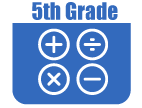Resources by Strand

Strand: MATHEMATICAL PRACTICES (5.MP)
The Standards for Mathematical Practice in Fifth Grade describe mathematical habits of mind that teachers should seek to develop in their students. Students become mathematically proficient in engaging with mathematical content and concepts as they learn, experience, and apply these skills and attitudes.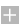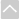Strand: OPERATIONS AND ALGEBRAIC THINKING (5.OA)
Write and interpret numerical expressions (Standards 5.OA.1–2)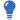Core Guide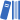OER Curriculum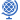Instructional TasksReview Activitiy

Standard 5.OA.1
Use parentheses, brackets, or braces in numerical expressions, and evaluate expressions with these symbols.

Standard 5.OA.2
Write and interpret simple numerical expressions.

1. Write simple expressions that record calculations with numbers. For example, use 2 x (8+7) to express the calculation "add 8 and 7, then multiply by 2."
2. Interpret numerical expressions without evaluating them. For example, use conceptual understanding of multiplication to interpret 3 x (18939 + 921) as being three times as large as 18932 + 921 without calculating the indicated sum or product.
Analyze patterns and relationships (Standard 5.OA.3)Core GuideOER CurriculumInstructional TasksReview Activitiy

Standard 5.OA.3
Generate two numerical patterns using two given rules. Identify apparent relationships between corresponding terms. Form ordered pairs consisting of corresponding terms from the two patterns, and graph the ordered pairs on a coordinate plane. For example, given the rule "add 3" and the starting number 0, and given the rule "add 6" and the starting number 0, generate terms in the resulting sequences, and observe that the terms in one sequence are twice the corresponding terms in the other sequence. Explain informally why this is so.Strand: NUMBER AND OPERATIONS IN BASE TEN (5.NBT)
Understand the place value system (Standards 5.NBT.1–4)Core GuideOER CurriculumInstructional TasksReview Activitiy

Standard 5.NBT.1
Recognize that in a multi-digit number, a digit in one place represents 10 times as much as it represents in the place to its right and 1/10 of what it represents in the place to its left.

Standard 5.NBT.2
Explain patterns in the number of zeros of the product when multiplying a number by powers of 10, and explain patterns in the placement of the decimal point when a decimal is multiplied or divided by a power of 10. Use whole-number exponents to denote powers of 10.

Standard 5.NBT.3
Read, write, and compare decimals to thousandths.

1. Read and write decimals to thousandths using base-ten numerals, number names, and expanded form. For example, 347.392 = 3 x 100 + 4 x 10 + 7 x 1 + 3 x (1/10) + 9 x (1/100) + 2 x (1/1000).
2. Compare two decimals to thousandths based on meanings of the digits in each place, using >, =, and < symbols to record the results of comparisons.

Standard 5.NBT.4
Use place value understanding to round decimals to any place.

Perform operations with multidigit whole numbers and with decimals to hundredths (Standards 5.NBT.5–7)Core GuideOER CurriculumInstructional TasksReview Activitiy

Standard 5.NBT.5
Fluently multiply multi-digit whole numbers using the standard algorithm.

Standard 5.NBT.6
Find whole-number quotients of whole numbers with up to four-digit dividends and two- digit divisors, using strategies based on place value, the properties of operations, and/or the relationship between multiplication and division. Illustrate and explain the calculation by using equations, rectangular arrays, and/or area models.

Standard 5.NBT.7
Add, subtract, multiply, and divide decimals to hundredths, using concrete models or drawings and strategies based on place value, properties of operations, and/or the relationship between addition and subtraction; relate the strategy to a written method and explain the reasoning used. In this standard, dividing decimals is limited to a whole number dividend with a decimal divisor or a decimal dividend with a whole number divisor. Compare the value of the quotient on the basis of the values of the dividend and divisor.Strand: NUMBER AND OPERATIONS - FRACTIONS (5.NF)
Use equivalent fractions as a strategy to add and subtract fractions (Standards 5.NF.1–2)Core GuideOER CurriculumInstructional TasksReview Activitiy

Standard 5.NF.1
Add and subtract fractions with unlike denominators (including mixed numbers) by replacing given fractions with equivalent fractions in such a way as to produce an equivalent sum or difference of fractions with like denominators. For example, 2/3 + 5/4 = 8/12 + 15/12 = 23/12. (In general, a/b + c/d = (ad + bc)/bd.)

Standard 5.NF.2
Solve real-world problems involving addition and subtraction of fractions referring to the same whole, including cases of unlike denominators by, for example, using visual fraction models or equations to represent the problem. Use benchmark fractions and number sense of fractions to estimate mentally and assess the reasonableness of answers. For example, recognize 2/5 + 1/2 = 3/7 as an incorrect result, by observing that 3/7 < 1/2.

Apply and extend previous understandings of multiplication and division to multiply and divide fractions (Standards 5.NF.3–7)Core GuideOER CurriculumInstructional TasksReview Activitiy

Standard 5.NF.3
Interpret a fraction as division of the numerator by the denominator (a/b = a ÷ b). Solve real-world problems involving division of whole numbers leading to answers in the form of fractions or mixed numbers, through the use of visual fraction models or equations to represent the problem. For example, interpret 3/4 as the result of dividing three by four, noting that 3/4 multiplied by four equals three, and that when three wholes are shared equally among four people each person has a share of size 3/4. If nine people want to share a 50-pound sack of rice equally by weight, how many pounds of rice should each person get? Between what two whole numbers does your answer lie?

Standard 5.NF.4
Apply and extend previous understandings of multiplication to multiply a fraction or whole number by a fraction.

1. Interpret the product (a/b) x q as a parts of a partition of q into b equal parts; equivalently, as the result of a sequence of operations a x q ÷ b using a visual fraction model. For example, use a fraction model to show (2/3) x 4 = 8/3, and create a story context for this equation. Do the same with (2/3) x (4/5) = 8/15. (In general, (a/b) x (c/d) = ac/bd.)
2. Find the area of a rectangle with fractional side lengths by tiling it with unit squares of the appropriate unit fraction side lengths, and show that the area is the same as would be found by multiplying the side lengths. Multiply fractional side lengths to find areas of rectangles, and represent fraction products as rectangular areas.

Standard 5.NF.5
Interpret multiplication as scaling.

1. Compare the size of a product to the size of one factor on the basis of the size of the other factor, without performing the indicated multiplication. For example, the products of expressions such as 5 x 3 or ½ x 3 can be interpreted in terms of a quantity, three, and a scaling factor, five or ½. Thus in addition to knowing that 5 x 3 = 15, they can also say that 5 x 3 is five times as big as three, without evaluating the product. Likewise they see ½ x 3 as half the size of three.
2. Explain why multiplying a given number by a fraction greater than one results in a product greater than the given number (recognizing multiplication by whole numbers greater than one as a familiar case); explain why multiplying a given number by a fraction less than one results in a product smaller than the given number; and relate the principle of fraction equivalence. For example, 6/10 = (2x3)/(2x5). In general, a/b = (n x a)/(n x b) has the effect of multiplying a/b by one.

Standard 5.NF.6
Solve real-world problems involving multiplication of fractions and mixed numbers, for example, by using visual fraction models or equations to represent the problem.

Standard 5.NF.7
Apply and extend previous understandings of division to divide unit fractions by whole numbers and whole numbers by unit fractions. Use strategies to divide fractions by reasoning about the relationship between multiplication and division. Division of a fraction by a fraction is not a requirement at this grade.

1. Interpret division of a unit fraction by a non-zero whole number, and compute such quotients. For example, create a story context for (1/3) ÷ 4, and use a visual fraction model to show the quotient. Use the relationship between multiplication and division to explain that (1/3) ÷ 4 = 1/12 because (1/12) x 4 = 1/3.
2. Interpret division of a whole number by a unit fraction, and compute such quotients. For example, create a story context for 4 ÷ (1/5), and use a visual fraction model to show the quotient. Use the relationship between multiplication and division to explain that 4 ÷ (1/5) = 20 because 20 x (1/5) = 4.
3. Solve real-world problems involving division of unit fractions by non-zero whole numbers and division of whole numbers by unit fractions, for example, by using visual fraction models and equations to represent the problem. For example, how much chocolate will each person get if three people share 1/2 lb. of chocolate equally? How many 1/3-cup servings are in two cups of raisins?Strand: MEASUREMENT AND DATA (5.MD)
Convert like measurement units within a given measurement system (Standard 5.MD.1)Core GuideOER CurriculumInstructional TasksReview Activitiy

Standard 5.MD.1
Convert among different-sized standard measurement units within a given measurement system (for example, convert 5 cm to 0.05 m); use these conversions in solving multi-step, real-world problems.

Represent and interpret data (Standard 5.MD.2)Core GuideOER CurriculumInstructional TasksReview Activitiy

Standard 5.MD.2
Make a line plot to display a data set of measurements in fractions of a unit (halves, quarters, eighths). Use operations on fractions for this grade to solve problems involving information presented in line plots. For example, given graduated cylinders with different measures of liquid in each, find the amount of liquid each cylinder would contain if the total amount in all the cylinders were redistributed equally.

Understand concepts of geometric measurement and volume, as well as how multiplication and addition relate to volume (Standard 5.MD.3-5)Core GuideOER CurriculumInstructional TasksReview Activitiy

Standard 5.MD.3
Recognize volume as an attribute of solid figures and understand concepts of volume measurement.

1. A cube with side length one unit, called a "unit cube," is said to have "one cubic unit" of volume, and can be used to measure volume.
2. A solid figure which can be packed without gaps or overlaps using n unit cubes is said to have a volume of n cubic units.

Standard 5.MD.4
Measure volumes by counting unit cubes, using cubic cm, cubic in., cubic ft., and improvised units.

Standard 5.MD.5
Relate volume to the operations of multiplication and addition and solve real-world and mathematical problems involving volume.

1. Find the volume of a right rectangular prism with whole-number side lengths by packing it with unit cubes, and show that the volume is the same as would be found by multiplying the edge lengths, equivalently by multiplying the height by the area of the base. Represent threefold whole-number products as volumes, for example, to represent the associative property of multiplication.
2. Apply the formulas V = l x w x h and V = b x h for rectangular prisms to find volumes of right rectangular prisms with whole-number edge lengths in the context of solving real-world and mathematical problems.
3. Recognize volume as additive. Find volumes of solid figures composed of two nonoverlapping right rectangular prisms by adding the volumes of the non-overlapping parts, applying this technique to solve real-world problems.Strand: GEOMETRY (5.G)
Graph points on the coordinate plane to solve real-world and mathematical problems in quadrant one (Standards 5.G.1–2)Core GuideOER CurriculumInstructional TasksReview Activitiy

Standard 5.G.1
Compose and understand the coordinate plane.

1. Use a pair of perpendicular number lines, called axes, to define a coordinate system, with the intersection of the lines (the origin) arranged to coincide with the zero on each line and a given point in the plane located by using an ordered pair of numbers, called its coordinates.
2. Using quadrant one on the coordinate plane, understand that the first number in a coordinate pair indicates how far to travel from the origin in the direction of the horizontal axis, and the second number indicates how far to travel in the direction of the vertical axis, with the convention that the names of the two axes and the coordinates correspond (x-axis and x-coordinate, y-axis and y-coordinate).

Standard 5.G.2
Represent real-world and mathematical problems by graphing points in the first quadrant of the coordinate plane, and interpret coordinate values of points in the context of the situation.

Classify two-dimensional figures into categories based on their properties. (Standards 5.G.3–4)Core GuideOER CurriculumInstructional TasksReview Activitiy

Standard 5.G.3
Understand that attributes belonging to a category of two-dimensional figures also belong to all subcategories of that category. For example, all rectangles have four right angles and all squares are rectangles, so all squares have four right angles.

Standard 5.G.4
Classify two-dimensional figures in a hierarchy based on properties.The Online Core Resource pages are a collaborative project between the Utah State Board of Education and the Utah Education Network. If you would like to recommend a high quality resource, contact Trish French (Elementary) or Lindsey Henderson (Secondary). If you find inaccuracies or broken links contact resources@uen.org.